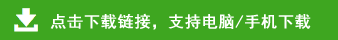# 北师大版小学三年级数学上册期末检测题（附答案）

北师大版小学三年级数学上册期末检测题（附答案）部分内容预览

一、计算题：
1、口算：
4×12=     600÷3=      32×3=      4×16=     2×40=
60+8=      150÷5=     240÷8=     25×4=    630÷7=
302×3=    300÷6=      48÷4=      17×5=     16×5=
4×50=     26÷2=      26×2=      48÷4=       57+8=
9+6×3=    5+4×7=      8÷2+9=      8+5×6=
2、竖式计算。（带*的要验算）
420×7=       235×4=        605×8=        850×2=
902÷6=       *918÷9=       447÷3=        *817÷4=
3、脱式计算
35+95÷5      68×6－356      （345+15）÷9
=                   =                    =
=                  =                     =
798÷7÷6       624÷6×5         819÷（3×3）
=                   =                    =
=                   =                     =
二、选择正确的答案填空：
1、甲的周长与乙的周长 （     ）
A.甲长B.乙长C.同样长
2、下面图形周长比较（        ）
A.A长　B.B长　C.一样长
3、用5个边长为1厘米的正方形拼成下面的图形．周长较长的是(     )
*4、一个长方形的周长是24厘米, 如果把它平均剪成两个正方形, 每个正方形的周长是（     ）厘米 。
A、12               B、14              C、16
三、完成下面的题。
1、描出右面图形的周长。
2、小朋友用一根3米的绳子，正好圈了一个一个象上面的蝴蝶，那个蝴蝶的周长是（      ）米。
3、一个正方形的周长是124厘米，它的边长是（         ）。
4、我们可以用：“商×除数+余数=被除数”来（        ）除法计算算得对不对。
5、25×4=（     ）÷5=（     ）×5=（      ）+5=（      ）
6、不计算判断下面的商是几位。
582÷4         587÷8            431÷6             935÷3
（   ）位       （   ）位       （   ）位          （   ）位